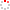#CodeStepByStep## partitionable

Language/Type: C++ dynamic programming

Write a function named `partitionable` that uses dynamic programming to discover whether a vector can be partitioned into two sub-lists of equal sum. Your function should accept a reference to vector of integers as a parameter, and return `true` if the given list can be partitioned equally, and `false` if not.

For example, the vector `{1, 2, 3}` can be split into `{1, 2}` and `{3}`, both of which have a sum of 3. The vector `{1, 2, 3, 4, 6}` can be split into `{1, 3, 4}` and `{2, 6}`, both of which have a sum of 8. For the vector `{2, 1, 8, 3}`, there is no way to split the vector into two sub-vectors whose sum is equal. The table below indicates various possible contents for a vector, and the value that would be returned by the call of your function:

Vector Contents Value Returned Reasoning
`{1, 2, 3, 4, 6}` `true` `{1, 3, 4}` and `{2, 6}`
`{1, 2, 3}` `true` `{1, 2}` and `{3}`
`{2, 1, 8, 3}` `false`
`{8, 8}` `true` `{8}` and `{8}`
`{42}` `false`
`{}` `true` `{}` and `{}`

The key constraint of this problem is that you must solve it using a bottom-up dynamic programming approach. Do not use recursion. Your solution must use dynamic programming instead. You are allowed to construct any data structures (array, vector, set, map, etc.) necessary to store the data for your dynamic programming algorithm. You may assume that none of the values in the vector are negative.

Type your C++ solution code here:

This is a function exercise. Write a C++ function as described. Do not write a complete program; just the function(s) above.